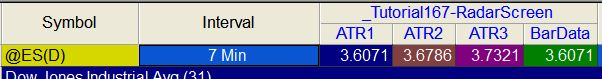# Tutorial 167 | Using a Price Series Provider to calculate the Average True Range

Tutorial 167 is a TradeStation EasyLanguage tutorial that demonstrates how to calculate the Average True Range (ATR) using a Price Series Provider (PSP).

The technique could be used, for example, to calculate the ATR for a 9 minute interval span, but applied to a 5 minute chart or to apply several ATRs for different bar intervals on a single row in RadarScreen.

The tutorial also shows why the ATR cannot be calculated by using the standard TradeStation function with a PSP.

All the examples in this tutorial use a minute IntervalType.

Make sure that the chart time is set to local (in order to match the PSP).

WARNING: on some versions of TradeStation synchronization issues between the chart data and the equivalent price series provider data have been observed. With this tutorial this will cause the PSP based plot to not line up precisely with a plot based on the data feed.

### Tutorial 167 video overview

In the second video I use syntax  from _Tutorial 167 to create new program (_Tutorial167-RadarScreen). In this program three PSPs are used to calculate three ATRs each with a different bar intervals. Each interval length is controlled by a user input:  IntSpan1, IntSpan2, and IntSpan3.

The program plots four columns as in the following image:ATR1 is the Average True Range based on an interval span of IntSpan1 (or 7 minutes in this case). ATR2 is the Average True Range based on an interval span of IntSpan2 (or 14 minutes in this case). ATR3 is the Average True Range based on an interval span of IntSpan3 (or 21 minutes in this case). The fourth column, BarData, calculates the ATR based on the data for this row, which has a 7 minite interval. Note that the values of ATR1 and BarData are the same.